Get instant live expert help with Excel or Google Sheets“My Excelchat expert helped me in less than 20 minutes, saving me what would have been 5 hours of work!”

#### Post your problem and you’ll get expert help in seconds.

Your message must be at least 40 characters
Our professional experts are available now. Your privacy is guaranteed.

# Excel Slope Function – Excelchat

The Slope Function in Excel calculates the slope of linear regression based on a supplied data set of x- and y- values.

Slope in Excel can be categorized under its statistical functions. In mathematical terms , Excel Slope determines the slope of any line between specified data points known as y’s and x’s values.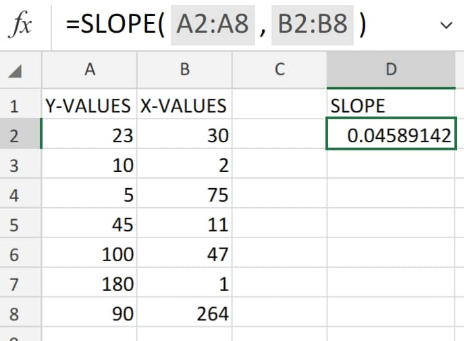Figure 1. of Slope Function in Excel

A linear regression slope in Excel is basically the vertical/horizontal distance between any two points on that line.

## Generic Formula

`=SLOPE(known_y's, known_x's)`

• Known_y’s =. An cell range or array of dependent numerical data points.
• Known_x’s =.An independent set of data.

## How to find slope of a line in Excel

We will now demonstrate the process of finding slope in Excel with these simple steps;

1. Let’s assume that we have we have two separate sets of data having known y’s and  x’s values; we start by collecting the information available to us inside our worksheet: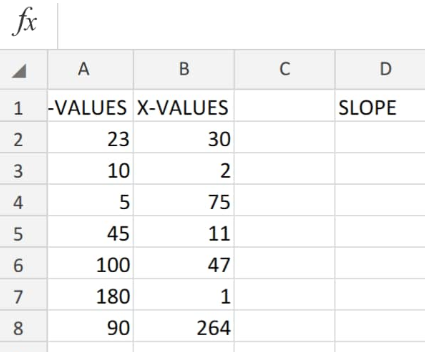Figure 2. of Slope Function in Excel

1. We will now calculate the slope of the y intercept Excel as well as the x intercept Excel from the data contained in columns A and B of our worksheet illustration by entering the following Excel Slope Formula into cell D2:

`=slope(A2:A8,B2:B8)`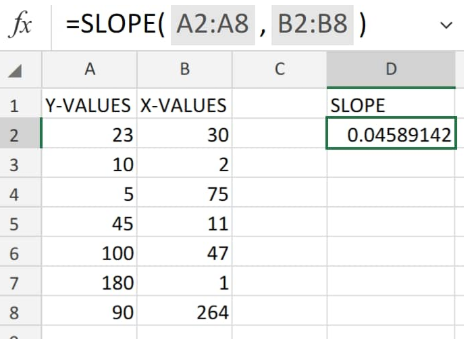Figure 3. of Slope Function in Excel

The Excel slope output will be 0.046 (approx.) as shown in our work table above.

In our next example, let’s say we have monthly data with known y and x values which we want to determine their slope in Excel.

See illustration below: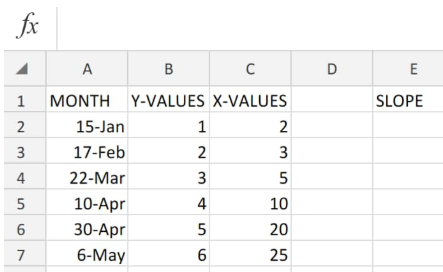Figure 4. of Slope Function in Excel

The slope formula for calculating the Excel Y intercept and Excel X intercept  in cell E2 above will be as follows:

`=SLOPE(B2:B7,C2:C7)`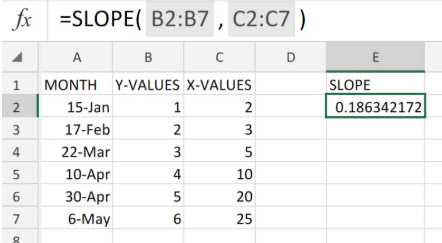Figure 5. of Slope Function in Excel

## Note

The slope Excel and intercept Excel algorithm are designed to search for only one answer.